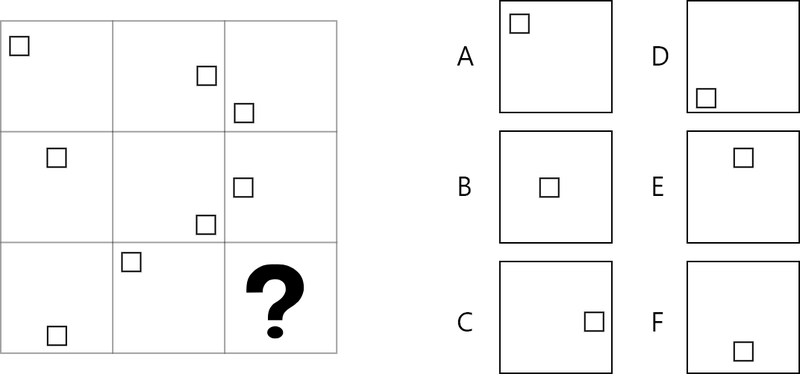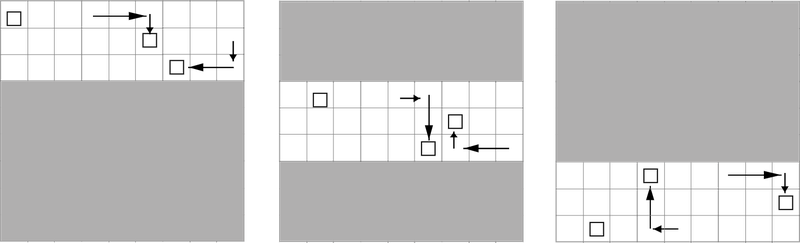# Abstract Matrix Explanation 1 - Movement Of The Figures

Matrix explanation - Movement of the figures

Many of the questions will be (partly) based on the location of the figures in the boxes. See for example the exercise below:From left to right, the square moves 3 spots clockwise along the edge. This is clearly visible in the figure below, where a grid has been added and per row you can see what the transformations are.Frequently occurring transformations are:

• In the horizontal plane, for example
• from right to left and back again
• each time a step to the right and at the end the figure starts again on the left
• each time a step to the left and at the end the figure starts again on the right, but then a row lower or higher.
• In the vertical plane, similar to the examples above.
• Along the edge of the matrix, clockwise or counter clockwise.
• Mirrored relative to, for example, the center.
• Two figures change places

You can study 3 example questions and then practice 3 exercises in which the movement of the figure is an important part of the question.